# Drone Patrol

An autonomous drone equipped with an AI to detect fire on an early stage and deliver first-aid kits to victims.

IntermediateFull instructions providedOver 8 days748

## Things used in this project

### Hardware components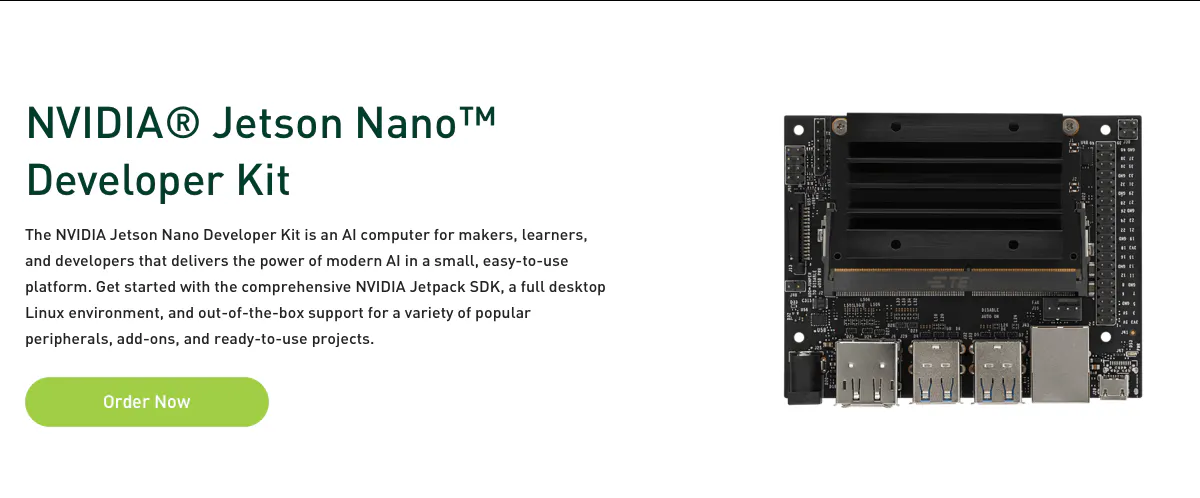NVIDIA Jetson Nano Developer Kit
×1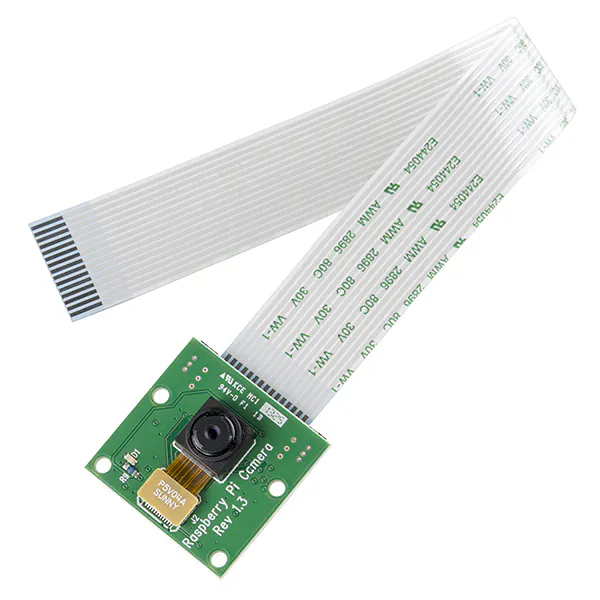Raspberry Pi Camera Module
×1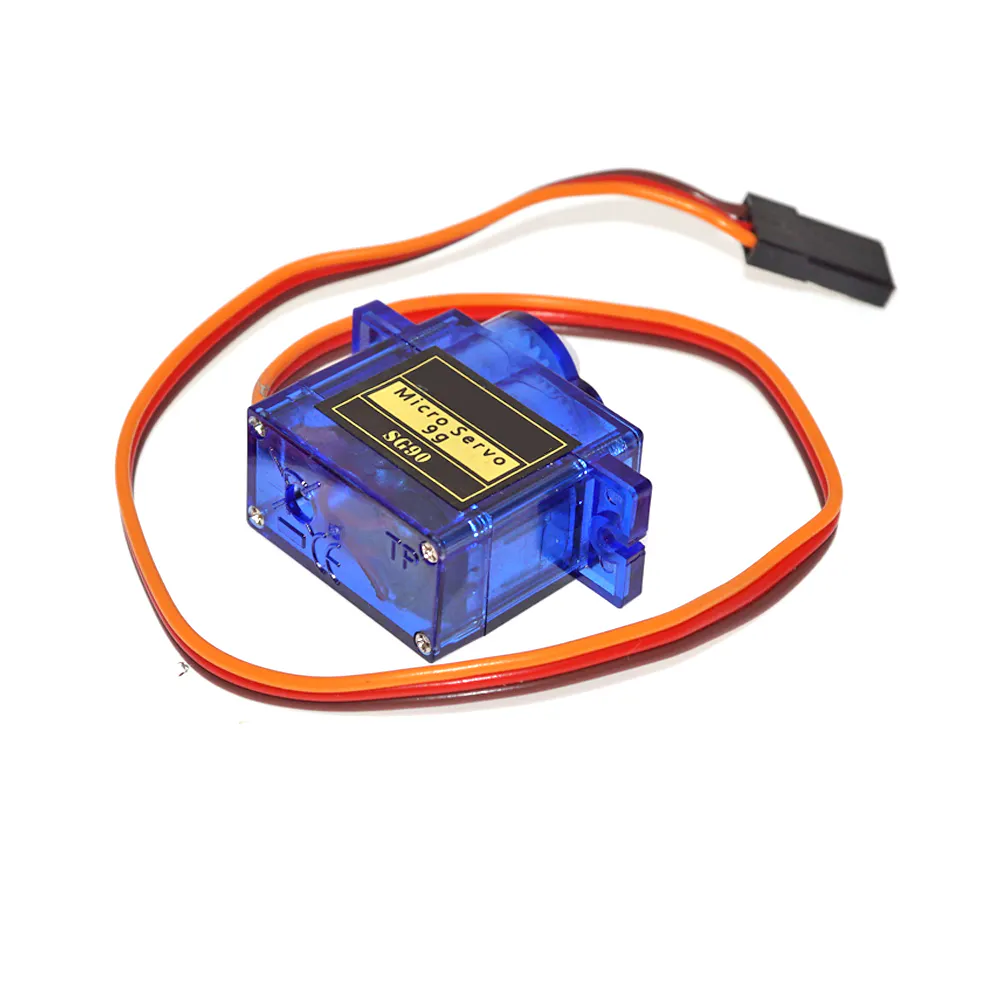SG90 Micro-servo motor
×1
 Wifi module
×1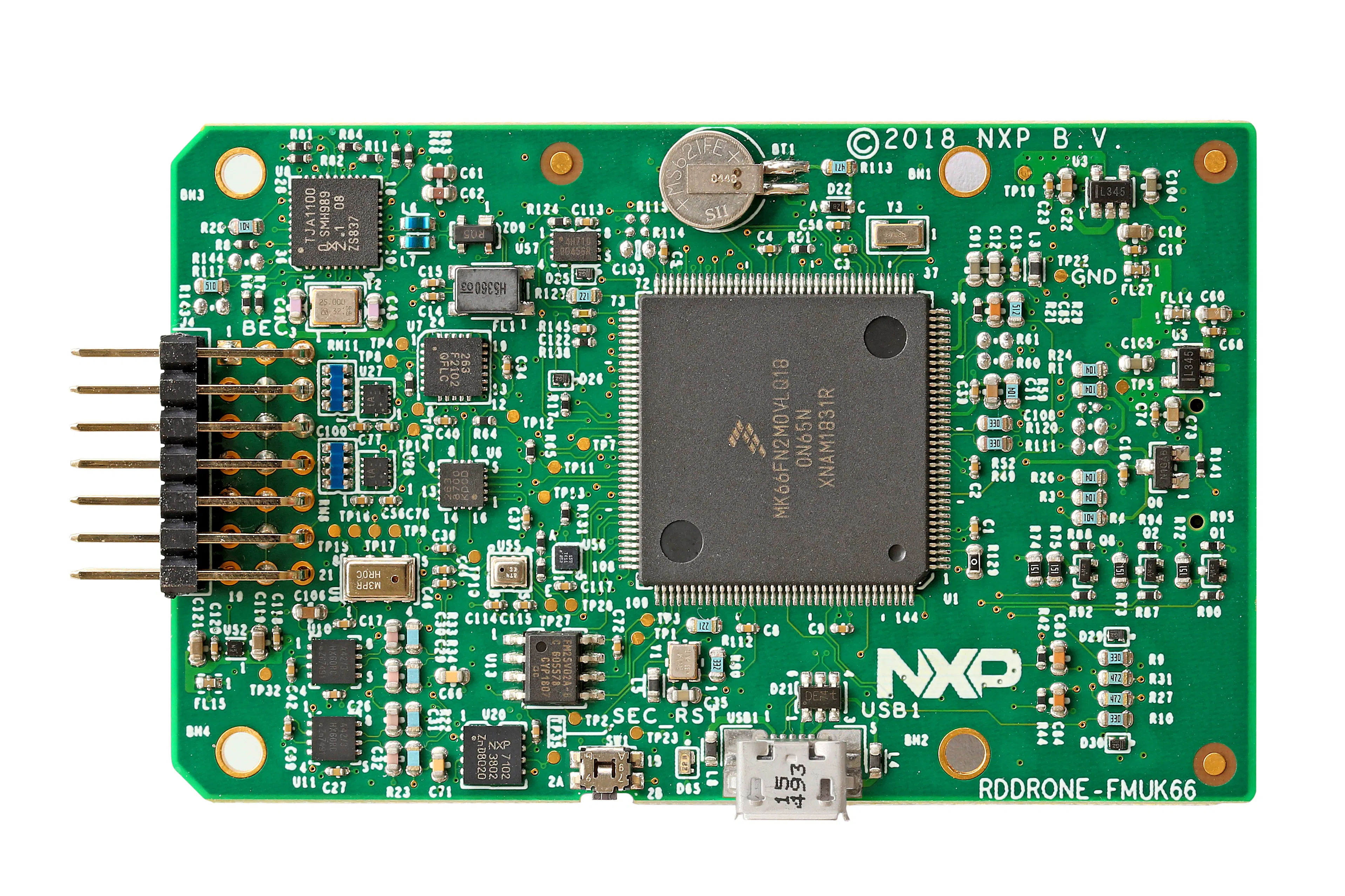NXP RDDRONE-FMUK66
×1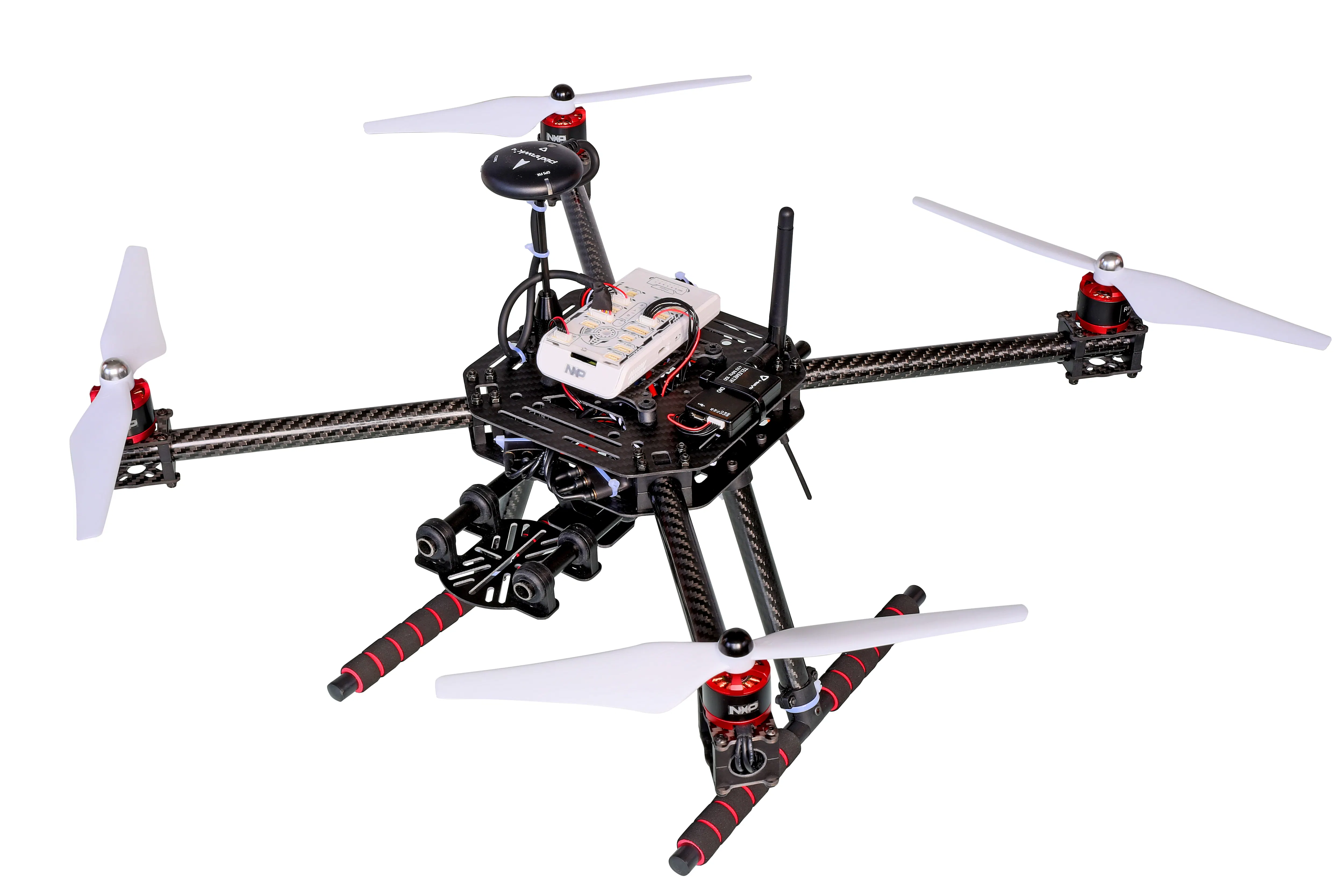NXP KIT-HGDRONEK66
×1

### Software apps and online servicesOpenCV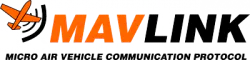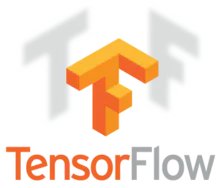TensorFlow

### Hand tools and fabrication machines3D Printer (generic)

## Schematics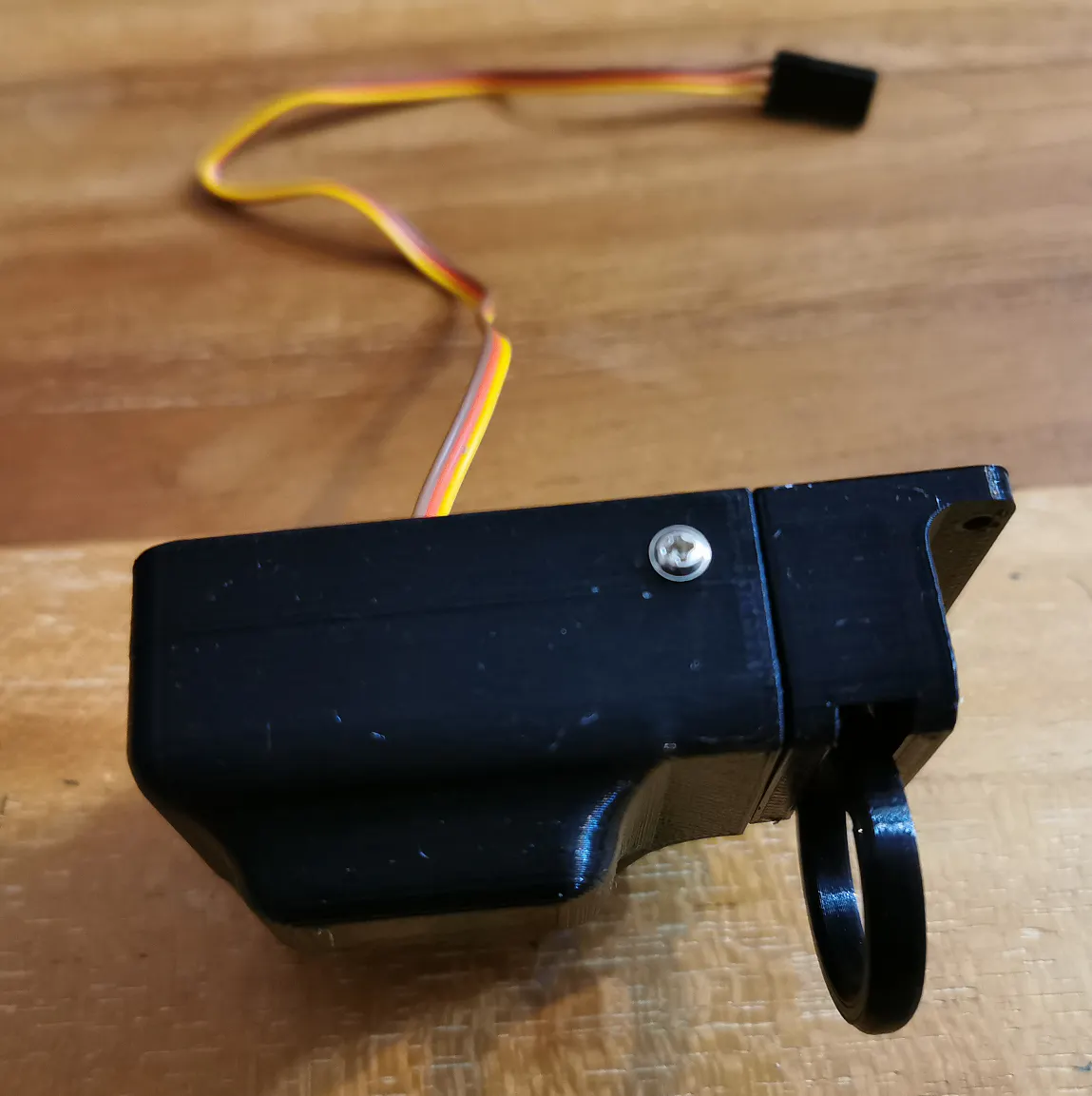## Code

### Drone Patrol Python code (Desktop version)

Python
```import cv2
import os
import sys
import math
import time

import tflearn
from tflearn.layers.core import *
from tflearn.layers.conv import *
from tflearn.layers.normalization import *
from tflearn.layers.estimator import regression

from dronekit import connect, VehicleMode, mavutil

def set_servo(servo_number, pwm_value):
pwm_value_int = int(pwm_value)

def my_new_fix_targets(message):
pass

#return a CNN tensorflow model
def construct_fireCNN (x,y):

network = tflearn.input_data(shape=[None, y, x, 3], dtype=tf.float32)

network = conv_2d(network, 64, 5, strides=4, activation='relu')

network = max_pool_2d(network, 3, strides=2)
network = local_response_normalization(network)

network = conv_2d(network, 128, 4, activation='relu')

network = max_pool_2d(network, 3, strides=2)
network = local_response_normalization(network)

network = conv_2d(network, 256, 1, activation='relu')

network = max_pool_2d(network, 3, strides=2)
network = local_response_normalization(network)

network = fully_connected(network, 4096, activation='tanh')
network = fully_connected(network, 4096, activation='tanh')
network = fully_connected(network, 2, activation='softmax')

# constuct final model

model = tflearn.DNN(network, checkpoint_path='fire',
max_checkpoints=1, tensorboard_verbose=2)

return model

connection_string = "/dev/ttyUSB0" #connection to Jetson Nano USB port
baud_rate = 115200
print(">>>> Connecting with the UAV <<<")
vehicle = connect(connection_string,baud_rate, wait_ready=True)     #- wait_ready flag hold the program untill all the parameters are been read
udp_conn = MAVConnection('udpin:192.168.0.22:15667', source_system=1) #open an incoming udp connection on Jetson Nano
vehicle._handler.pipe(udp_conn)
udp_conn.master.mav.srcComponent = 1  # needed to make QGroundControl work!
udp_conn.start()
udp_conn.fix_targets = my_new_fix_targets

################################################################################

if __name__ == '__main__':

################################################################################

while not vehicle.is_armable:
print("waiting to be armable")
time.sleep(1)

print("Arming motors")
vehicle.mode = VehicleMode("AUTO")
vehicle.armed = True

while not vehicle.armed: time.sleep(1)

# construct and display model

model = construct_fireCNN (224, 224)
print("Constructed Fire CNN ...")

################################################################################

# network input sizes

rows = 224
cols = 224

# display and loop settings

windowName = "HoverGames Challenge 1: Fight Fire with Flyers with NXP";
keepProcessing = True;

# load video from default camera

video = cv2.VideoCapture(0)

# create window

cv2.namedWindow(windowName, cv2.WINDOW_NORMAL);

# get video properties

width = int(video.get(cv2.CAP_PROP_FRAME_WIDTH));
height = int(video.get(cv2.CAP_PROP_FRAME_HEIGHT))
fps = video.get(cv2.CAP_PROP_FPS)
frame_time = round(1000/fps);

while (keepProcessing):

# start a timer (to see how long processing and display takes)

start_t = cv2.getTickCount();

# get video frame from camera

# re-size image to network input size and perform prediction

small_frame = cv2.resize(frame, (rows, cols), cv2.INTER_AREA)
output = model.predict([small_frame])

# label image based on prediction

if round(output) == 1:
cv2.rectangle(frame, (0,0), (width,height), (0,0,255), 50)
cv2.putText(frame,'FIRE',(int(width/16),int(height/4)),
cv2.FONT_HERSHEY_SIMPLEX, 2,(255,255,255),7,cv2.LINE_AA);
msg = vehicle.message_factory.statustext_encode(1,b'Alert Fire at : ' + str( vehicle.location.global_frame).encode())
udp_conn.master.mav.send(msg)
else:
cv2.rectangle(frame, (0,0), (width,height), (0,255,0), 50)
cv2.putText(frame,'CLEAR',(int(width/16),int(height/4)),
cv2.FONT_HERSHEY_SIMPLEX, 2,(255,255,255),7,cv2.LINE_AA);

# stop the timer and convert to ms. (to see how long processing and display takes)

stop_t = ((cv2.getTickCount() - start_t)/cv2.getTickFrequency()) * 1000;

# image display and key handling

cv2.imshow(windowName, frame);

# wait fps time or less depending on processing time taken (e.g. 1000ms / 25 fps = 40 ms)

key = cv2.waitKey(max(2, frame_time - int(math.ceil(stop_t)))) & 0xFF;
if (key == ord('x')):
keepProcessing = False;
elif (key == ord('f')):
cv2.setWindowProperty(windowName, cv2.WND_PROP_FULLSCREEN, cv2.WINDOW_FULLSCREEN);
```

## Credits

### Amine Amri

6 projects • 7 followers